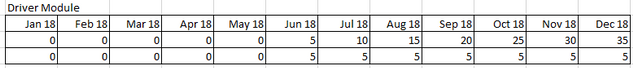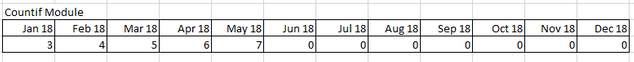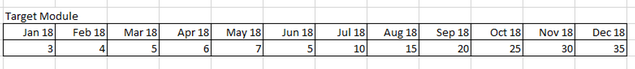# Circular Reference IssueHi Guys,

I need help in figuring out a workaround for Circular Reference. I recreated the modules the best I can and I hope its enough to understand.

See below:

This is the driver module and this is already working fine in Anaplan. Lets say Row 1 and Row 2 and then Time Dimension.This is the countif module, this also working fine in Anaplan.This is the Target Module. This is where the Circular reference is appearing.The formula here is IF Driver Module Row 1<=0 THEN Countif Module ELSE Countif  Row 1+ Driver Module Row 2

the logic here is when Driver Module Row 1 is valued at 0 then it should capture Countif Module Row 1 and if Driver Module Row 1 is more than 0 then it should capture Driver Module Row 2. This part of the formula has no problem IF Driver Module Row 1<=0 THEN Countif Module ELSE Countif  Row 1 but when I add + Driver Module Row 2 in the formula that is where the circular reference comes up.

Any idea? Thank you in advance.

Regards,

Tagged:

•It may help to split out the IF statement, it will definitely help reduce calculations as there are some repeated functions.

IF 'Row 7' < '(M) Actuals - Details (V)'.'SALES VELOCITY/MO. (NET)' THEN 0 ELSE D

where:
A = CUMULATE('(M) Actual Monthly - OPStat (V)'.'(B& FP) Units')
B = CUMULATE('(M) Actual Monthly - OPStat (V)'.'(R& w/ DP) Units')
C = A + B
D = IF C < '(M) Actuals - Details (V)'.'TOTAL NO. OF UNITS' THEN '(M) Actuals - Details (V)'.'SALES VELOCITY/MO. (NET)' ELSE '(M) Actuals - Details (V)'.'TOTAL NO. OF UNITS' - C

•Could you post the formulas you are using in your driver and countif module too? is there a dependency between these modules?

•Would it not be better to make the selection logic of which line item to use in the source module and then point to that.

I believe this would resolve the circular reference error if i'm understanding this correctly?

Thanks,

Usman

•Hi,

Appreciate your response. Here is the formula from the Drivers module.

Row 1 in the example.

'Row 6' * '(M) Actuals - Details (V)'.'SALES VELOCITY/MO. (NET)'

Row 6 is in the same module which results to a sequential number (1,2...55).

'(M) Actuals - Details (V)'.'SALES VELOCITY/MO. (NET)' - Is part of the assumption module which is inputted (Constant value 5).\\

Row 2 in the example

IF 'Row 7' < '(M) Actuals - Details (V)'.'SALES VELOCITY/MO. (NET)' THEN 0 ELSE IF CUMULATE('(M) Actual Monthly - OPStat (V)'.'(B& FP) Units') + CUMULATE('(M) Actual Monthly - OPStat (V)'.'(R& w/ DP) Units') < '(M) Actuals - Details (V)'.'TOTAL NO. OF UNITS' THEN '(M) Actuals - Details (V)'.'SALES VELOCITY/MO. (NET)' ELSE '(M) Actuals - Details (V)'.'TOTAL NO. OF UNITS' - (CUMULATE('(M) Actual Monthly - OPStat (V)'.'(B& FP) Units') + CUMULATE('(M) Actual Monthly - OPStat (V)'.'(R& w/ DP) Units'))

It basically adds the cumulative value of two line items in the FS report from Jan 13 to a specific date base on the drivers module. total number of units is 448 which is a formula.

Countif Module

IF '(M) Actual Monthly - Drivers (V)'.'Row 7' <= 0 THEN '(M) Actual GENCOM - (V)'.'Count Resi (Res,DP)'[SUM: 'Driver Module - Gencon (V)'.Period RS] ELSE '(M) Actual GENCOM - (V)'.'Count Resi (Res,DP)'[SUM: 'Driver Module - Gencon (V)'.Period RS]

(M) Actual GENCOM - (V)'.'Count Resi (Res,DP)'[SUM: 'Driver Module - Gencon (V)'.Period RS] = Is the formula to count units from the source module depending on the date (Driver Module - Gencon (V)'.Period RS).

Regards,